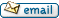•http://facebook.com/
•https://www.google.com/accounts/o8/id
•https://me.yahoo.com
engineering › fluids ›

# Reynolds

Evaluates the Reynolds number of given parameters.
Controller: CodeCogsContents
Private project under development, to help contact the author:C++
Excel

## Reynolds

 doubleReynolds( double diameter double velocity double density double viscosity )[inline]
Reynolds number, named after Osborne Reynolds who proposed it in 1883, is the ratio of inertial forces to viscous forces in a fluid. Besides other applications, it can be used to determine whether a flow inside a pipe is laminar, turbulent or in the so-called critical zone. The formula for this dimensionless measure is: where is the characteristic length, is the average velocity of the flow, is the weight density and is the absolute viscosity of the fluid.

It has been established that in the case of a fluid going through pipes, for Reynolds values less than 2000 the flow is laminar, for values greater than 4000 the flow is turbulent, while for numbers between 2000 and 4000 the flow becomes unpredictable. For this reason the domain from 2000 to 4000 is also called the "critical zone".

### Example 1

#include <stdio.h>
#include <codecogs/engineering/fluids/reynolds.h>

int main()
{
double Re = Engineering::Fluids::Reynolds(5, 10, 23, 0.45345);
printf("Re = %.13lf\n", Re);

if (Re < 2000) printf("Laminar flow.\n");
else if (Re > 4000) printf("Turbulent flow.\n");
else printf("Unpredictable flow (critical zone).\n");

return 0;
}

### Output

Re = 2536.1120299922814
Unpredictable flow (critical zone).

### References

The Engineering Division, Crane Co., "Flow of fluids through valves, fittings, and pipe", Chicago, 1957

### Parameters

 diameter characteristic length (ft) velocity mean velocity of flow (ft/s) density weight density of fluid (lb/ft) viscosity absolute viscosity of fluid (lb/ft s)

### Returns

The Reynolds number corresponding to the given parameters.

### Authors

Lucian Bentea (July 2006)
##### Source Code

This module is private, for owner's use only.

Not a member, then Register with CodeCogs. Already a Member, then Login.